In :
%matplotlib inline


# Data Analysis Examples¶

## Introduction¶

In this notebook, I'm going to demonstrate the process of data analysis in the real world using two relatively simple datasets. I will also try to explain how to apply the scientific method.

It is meant to be a supplement to the lecture "Python Crash Course" from the course "Data Science" in SoftUni.

The datasets used are properties of their respective owners.

## Example 1. Gas Consumption¶

### Background¶

The dataset represents data from 15 houses collected during a single day. The measured variables are:

• Temperature difference (inside - outside), averaged over the entire day, $\Delta T\ [^\circ \text{C}]$
• Gas consumption, $C\ [\text{kWh}]$

### Question¶

How are these quantities related?

### Data Exploration¶

The dataset is pretty small, so we can inspect it directly.

In :
import pandas as pd
import matplotlib
import matplotlib.pyplot as plt
import numpy as np
from scipy import stats
from scipy.stats import ttest_ind

In :
gas_data = pd.read_table("temp_gas.csv")
gas_data

Out:
temp_diff power
0 10.3 69.81
1 11.4 82.75
2 11.5 81.75
3 12.5 80.38
4 13.1 85.89
5 13.4 75.32
6 13.6 69.81
7 15.0 78.54
8 15.2 81.29
9 15.3 99.20
10 15.6 86.35
11 16.4 110.23
12 16.5 106.55
13 17.0 85.50
14 17.1 90.02

The data appears to be clean - there are no missing values. We cannot be sure if there are any erroneous values (such as "human errors"). We can check for outliers by plotting histograms or boxplots.

In :
matplotlib.rcParams["text.usetex"] = True

def plot_histogram(data, axis, xlabel, ylabel):
axis.hist(data, bins = 5, normed = 1)
axis.set_xlabel(xlabel)
axis.set_ylabel(ylabel)

f, (ax1, ax2) = plt.subplots(2, figsize = (5, 6))
plot_histogram(gas_data.temp_diff, ax1, r"$\Delta T\ [^{\circ} \mathrm{C}]$", r"$\mathrm{Frequency}$")
plot_histogram(gas_data.power, ax2, r"$C\ [\mathrm{kWh}]$", r"$\mathrm{Frequency}$")

f.tight_layout()
plt.show()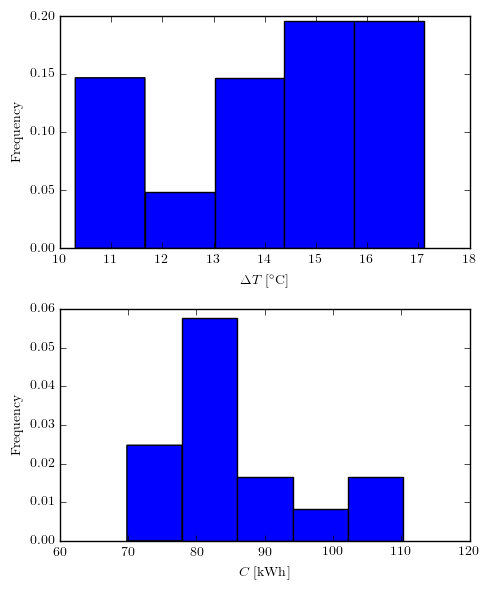Indeed, there are no outliers.

### Correlation¶

To see if there is any correlation between the two variables, we'll plot $C = f(T)$

In :
plt.scatter(gas_data.temp_diff, gas_data.power)
plt.xlabel(r"$\Delta T\ [^{\circ}\mathrm{C}]$")
plt.ylabel(r"$C\ [\mathrm{kWh}]$")
plt.show()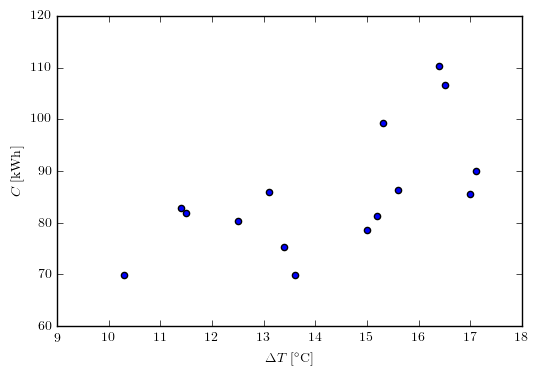There appears to be an upward trend. To see how strong it is, we will check the Pearson correlation coefficient.

In :
corr = np.corrcoef(gas_data.temp_diff, gas_data.power)
print("Correlation: " + str(corr))

Correlation: 0.626803947457


This indicates a positive correlation, that is, the gas consumption tends to increase when the temperature difference increases.

We can also add a trendline to the plot.

In :
a, b, r_value, p_value, std_err  = stats.linregress(gas_data.temp_diff, gas_data.power)
plt.scatter(gas_data.temp_diff, gas_data.power, label = None)
regr_label = r"$y = " + str(round(a, 2)) + "x + " + str(round(b,2)) + "$"
plt.plot(gas_data.temp_diff, gas_data.temp_diff * a + b, color = "red", linestyle = "--", label = regr_label)
plt.xlabel(r"$\Delta T\ [^{\circ}\mathrm{C}]$")
plt.ylabel(r"$C\ [\mathrm{kWh}]$")
plt.legend()
plt.show()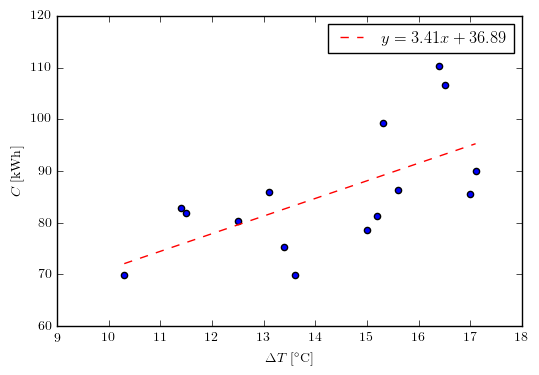## Example 2. Cloud Seeding¶

### Background¶

The dataset represents data from seeded and unseeded clouds. Each cloud was randomly chosen to be seeded or not. The values represent rainfall from the clouds $R [\text{mm}]$.

### Question¶

Does seeding increase rainfall?

### Data Exploration¶

Once again, the dataset is small enough, so let's just review it. We'll also rename the column names to make them "Pythonic".

In :
cloud_data = pd.read_table("clouds.csv", header = 0)
cloud_data.columns = ["unseeded", "seeded"]
cloud_data

Out:
unseeded seeded
0 1202.6 2745.6
1 830.1 1697.8
2 372.4 1656.0
3 345.5 978.0
4 321.2 703.4
5 244.3 489.1
6 163.0 430.0
7 147.8 334.1
8 95.0 302.8
9 87.0 274.7
10 81.2 274.7
11 68.5 255.0
12 47.3 242.5
13 41.1 200.7
14 36.6 198.6
15 29.0 129.6
16 28.6 119.0
17 26.3 118.3
18 26.1 115.3
19 24.4 92.4
20 21.7 40.6
21 17.3 32.7
22 11.5 31.4
23 4.9 17.5
24 4.9 7.7
25 1.0 4.1

Let's see how the values are distributed, again using histograms.

In :
def plot_histogram_clouds(data, axis, xlabel, ylabel, title):
axis.hist(data, bins = 10)
axis.set_xlabel(xlabel)
axis.set_ylabel(ylabel)
axis.set_title(title)

f, (ax1, ax2) = plt.subplots(2, figsize = (5, 6))
plot_histogram_clouds(cloud_data.unseeded, ax1, r"$R\ [\mathrm{mm}]$", "$N$", "$\mathrm{Unseeded\ clouds}$")
plot_histogram_clouds(cloud_data.seeded, ax2, r"$R\ [\mathrm{mm}]$", "$N$", "$\mathrm{Seeded\ clouds}$")

f.tight_layout()
plt.show()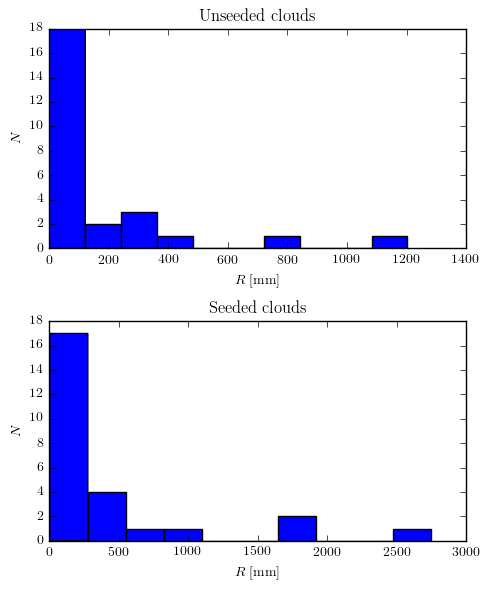The distributions may look the same but the units on the x axis are different. Looking at it, we can see that the seeded clouds have a much greater range.

To see the two distributions better, we can plot them together.

In :
f, ax = plt.subplots(figsize = (5, 6))
bin_width = 200
unseeded_bins = np.arange(min(cloud_data.unseeded), max(cloud_data.unseeded) + bin_width, bin_width)
seeded_bins = np.arange(min(cloud_data.seeded), max(cloud_data.seeded) + bin_width, bin_width)
ax.hist(cloud_data.unseeded, bins = unseeded_bins, alpha = 0.7, label = "$\mathrm{unseeded}$")
ax.hist(cloud_data.seeded, bins = seeded_bins, alpha = 0.7, label = "$\mathrm{seeded}$")
ax.set_xlabel("$R\ [\mathrm{mm}]$")
ax.set_ylabel("$N$")
ax.legend()

f.tight_layout()
plt.show()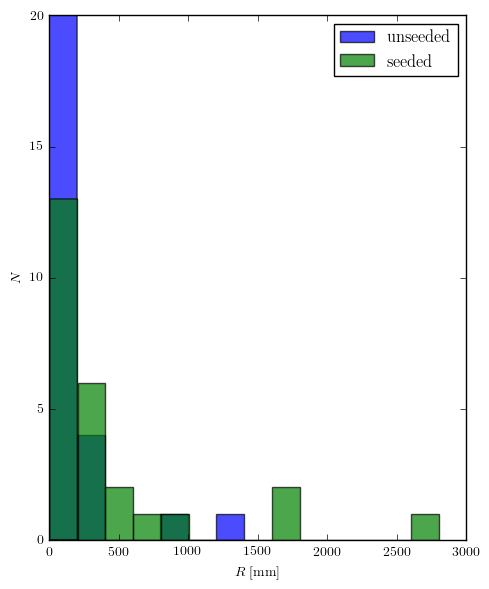Now we can see that the two distributions have very different ranges. We have to normalize these ranges in order to get meaningful results. The model we're going to run also requires normalized ranges.

A data transformation we can try is logarithm. It has the property of "shrinking" vast spans of data.

In :
cloud_data["log_unseeded"] = np.log(cloud_data.unseeded)
cloud_data["log_seeded"] = np.log(cloud_data.seeded)

f, ax = plt.subplots(figsize = (5, 6))
bin_width = 0.5
log_unseeded_bins = np.arange(min(cloud_data.log_unseeded), max(cloud_data.log_unseeded) + bin_width, bin_width)
log_seeded_bins = np.arange(min(cloud_data.log_seeded), max(cloud_data.log_seeded) + bin_width, bin_width)
ax.hist(cloud_data.log_unseeded, bins = log_unseeded_bins, alpha = 0.7, label = "$\mathrm{unseeded}$")
ax.hist(cloud_data.log_seeded, bins = log_seeded_bins, alpha = 0.7, label = "$\mathrm{seeded}$")
ax.set_xlabel("$\ln(R)$")
ax.set_ylabel("$N$")
ax.legend()

f.tight_layout()
plt.show()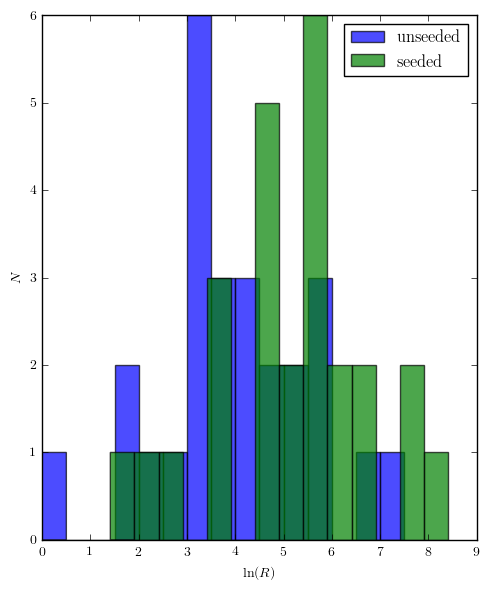Now the two distributions are both comparable (have similar ranges) and similar to normal. We can see that the seeded clouds appear to have more rainfall. We can see this better in a boxplot.

In :
f, ax = plt.subplots(figsize = (5, 6))
ax.boxplot([cloud_data.log_unseeded, cloud_data.log_seeded])
ax.set_xticklabels(["$\mathrm{unseeded}$", "$\mathrm{seeded}$"])
ax.set_ylabel("$\ln(\mathrm{R})$")

plt.show()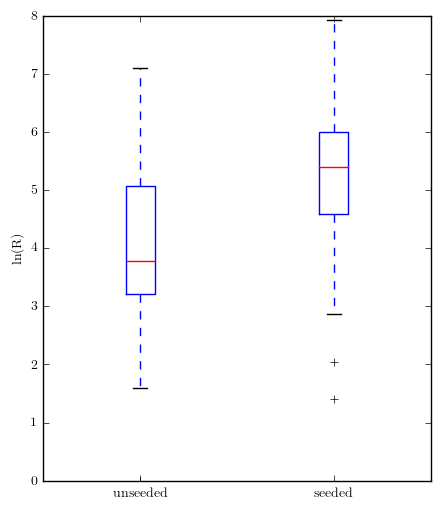We can definitely see that the distributions are different and the seeded clouds appear to rain more.

### Significance¶

A final question remains - is the result significant? That is, how likely is it that the difference we observe is random? We have to perform a t-test. Let's check for $H_0$ = "Rainfall from seeded clouds is not larger" and $\alpha = 0.05$.

In :
p_value = ttest_ind(cloud_data.log_unseeded, cloud_data.log_seeded).pvalue
# A detail here is that this p-value is for the "two-tailed" t-test but we only want to see
# whether one value is larger than the other - a "one-tailed" test
print("p-value: " + str(round((p_value / 2) * 100, 4)) + "%")

p-value: 0.7041%


The p-value is less than $\alpha$, so we can reject $H_0$. We can even make a stronger claim - that $\alpha = 0.01$ is also valid.

So, in conclusion, we can say that seeded clouds produce significantly more rain.

Note: These two values of $\alpha$ are accepted as standards.